Kernel Average Misorientation (KAM) edit page

The kernel average misorientation (KAM) is a measure of local grain misorientation that is usually derived from EBSD data. For formaly defining the KAM we denote by $$o_{i,j}$$ the orientations at pixel position $$(i,j)$$ and by $$N(i,j)$$ the set of all neighboring pixels. Then the kernel average misorientation $$\mathrm{kam}_{i,j}$$ at pixel position $$(i,j)$$ is defined as

$\mathrm{KAM}_{i,j} = \frac{1}{|N(i,j)|}\sum_{(k,l) \in N(i,j)} \omega(o_{i,j}, o_{k,l})$

Here $$N(i,j)$$ denotes the number of all neighboring pixels taking into account and $$\omega(o_{i,j}, o_{k,l})$$ the disorientation angle between the orientation $$o_{ij}$$ in the center and the neighbouring orientation $$(o_{k,l})$$. The specific choice of the set $$N(i,j)$$ of neighboring pixels is crucial for the compution of the KAM. Most commonly the following additional constrains are made

• consider neighbors up to order $$n$$, e.g. $$n=1,2,3,\ldots$$
• consider only neighbors belonging to the same grain
• consider only neighbors with a misorientation angle smaller than a threshold angle $$\delta$$

In the case of sqaure and hexagonal grids the order of neighbors is illustrated below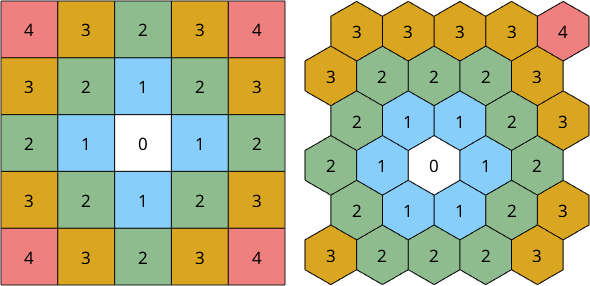## A Deformed Ferrite Specimen

Let us demonstrate the computation of the KAM at the example of a deformed Ferrite specimen. Lets import the data first and reconstruct the grain structure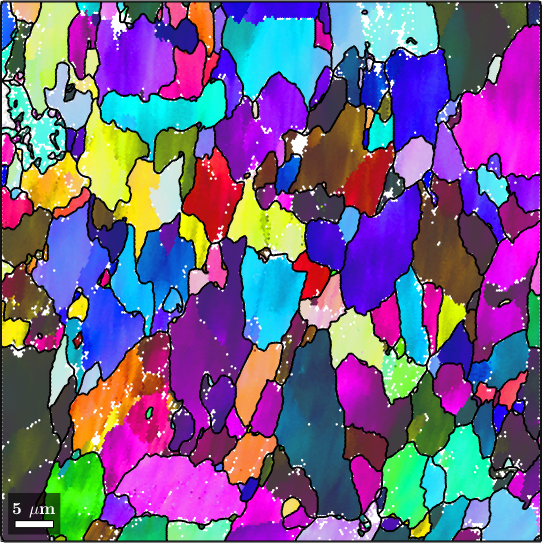Although MTEX allows the computation of the KAM from arbitrarily sampled EBSD maps the algorithms are much faster an memory efficient if the maps are measured on regular hexagonal or rectangular grid - as it is standard in most applications. The command gridify makes MTEX aware of such an underlying regular measurement grid.

The kernel average misorientation is computed by the command ebsd.KAM. As all MTEX commands it return the mean disorientation angle in radiant. Hence, dividing by the constant degree gives the result in degree.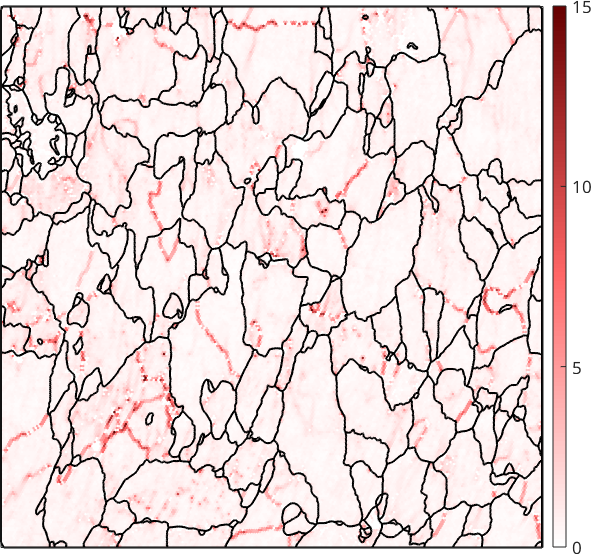When computed with default parameters in MTEX neighbors up to order 1 are considered and no threshold angle $$\delta$$ is applied. If grains have been reconstructed and the property ebsd.grainId has been set (as we did above) only misorientations within the same grain are considered. As a consequence the resulting KAM map is dominated by the orientation gradients at the subgrain boundaries.

Specifying a reasonable small theshold angle $$\delta=2.5^{\circ}$$ the subgrain boundaries can be effectively removed from the KAM.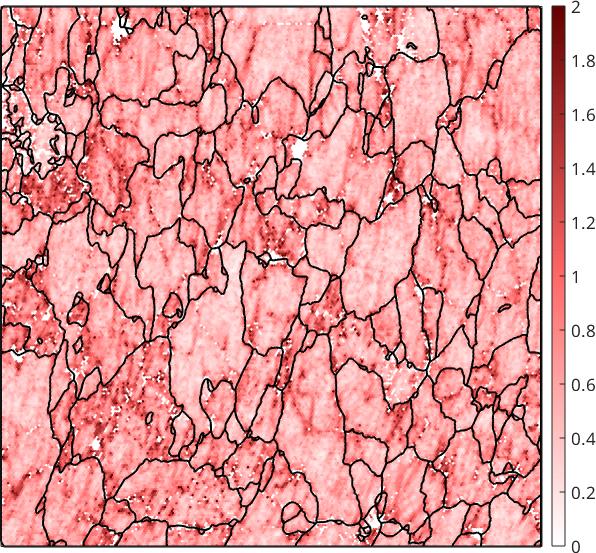Unfortunately, the remaining KAM becomes very sensitve to measurement errors and is often very noisy. The noise can be reduced by considering heigher order neighbors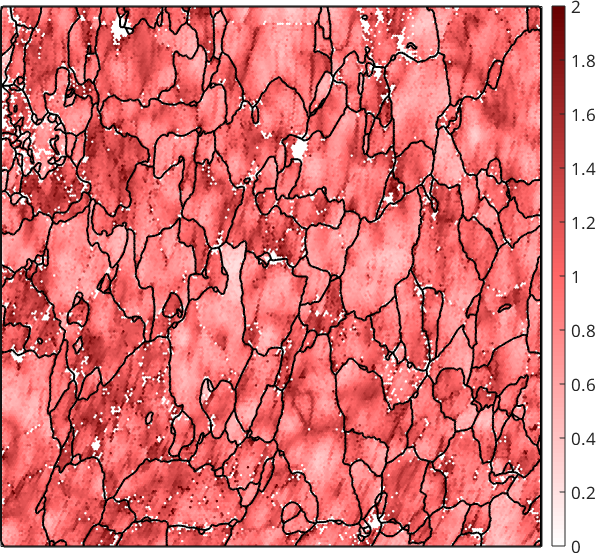Although this reduced noise it also smoothes away local dislocation structures. A much more effective way to reduce the effect of measurement errors to the kernel average misorientation is to denoise the EBSD map first and compute than the KAM from the first order neighbors.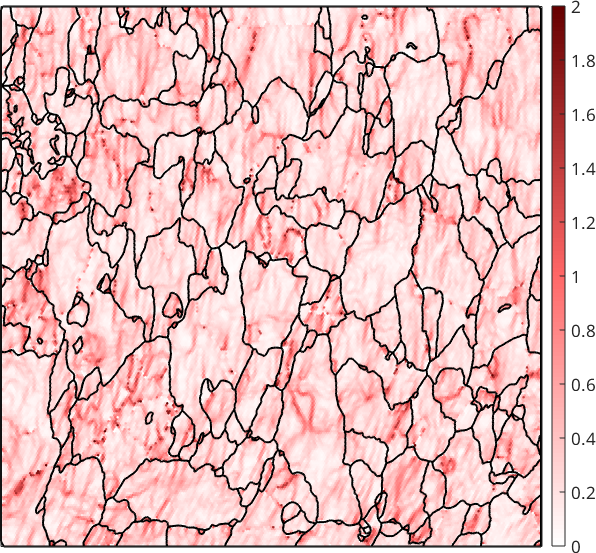We observe that the KAM is not longer related to subgrain boundaries and nicely revalves local dislocation structures of the deformed material.

## Some helper functions

The functions below where only used to generate the neighborhood pictures of the first paragraph